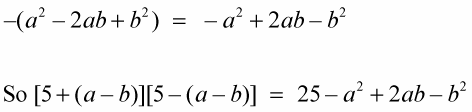##### Selling All-in-One For DummiesWhen distributing binomials over other terms, knowing how to find the sum and difference of the same two terms is a handy shortcut. The sum of any two terms multiplied by the difference of the same two terms is easy to find and even easier to work out — the result is simply the square of the two terms. The middle term just disappears because a term and its opposite are always in the middle.

If you encounter the same two terms and just the sign between them changes, rest assured that the result is the square of those two terms. The second term will always be negative, as in the example,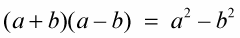Example 1: (x – 4)(x + 4)

You can use the shortcut to do these special distributions.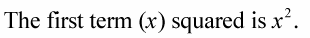The second term will always be negative, and a perfect square like the first term: (–4)(+4) = –16.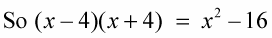Example 2: (ab – 5)(ab + 5)

Try the same easy process — multiplying the sum of two terms with their difference — with this slightly more complicated, variable term.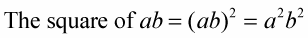The second term is negative, and a perfect square like the first term: 5 = –25.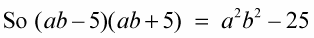Example 3: [5 + (ab)][5 – (ab)]

This example offers you a chance to work through the sum and difference of various groupings.

The square of 5 = 25

The second term is negative, and a perfect square like the first term: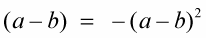Square the binomial and distribute the negative sign, which looks like this: